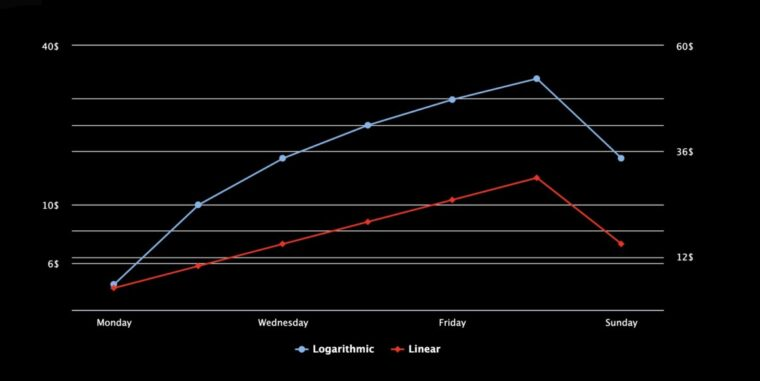# When should you use logarithmic or linear scales in charts?In the previous article dynamically switch between linear and log axis scale using stock tools GUI, you learned how to switch from a logarithmic to a linear interactive scale chart. In this article, I will cover the main differences between a linear scale chart and a logarithmic scale chart, and when to use each scale.

## What is a logarithmic scale?

A logarithmic scale chart is a chart where the value between two consecutive points on the y-Axis changes with a distinct pattern. The pattern is based on the following equation y=log b(10), where y is the power (exponent) that b is raised to in order to get x; in other words, the logarithm is the inverse function to exponentiation.

## When using a logarithmic chart?

A logarithmic scale is mainly used to display data with expansive value disparity. For instance, a logarithmic scale can easily render values from 10 to 100000 on the same chart. In contrast, if you use any other conventional chart, such as a simple line series with a linear axis, you will not notice details correlating with the smallest values, which could lead to misinterpretation of the data set.
The use of logarithmic scales are essential in the financial sector to highlight movement in relation to the percent change; it sounds complicated, let me explain this concept through an example:

The chart below visualizes dummy commodity price changes over a week using a logarithmic scale chart base 10.

The chart above shows that the commodity’s price rises \$5.00 from Monday to Tuesday and another \$5.00 from Thursday to Friday. However, the distance of the first movement (represented by the red plot band) is two times larger than the second movement (represented by the yellow plot band). The reason is that the logarithmic scale highlights the percent change between the consecutive values. The first movement ranges from \$5.00 to \$10.00, which is 50%, whereas the second movement, which ranges from \$20.00 to \$25.00, is just 25%.
By now, I think you get it that logarithmic charts are excellent to spot values’ percentages rather than the values’ differences. If you are in the financial sector, this chart can be beneficial to know when to reach a buy or sell target.

## What is a linear scale?

Contrary to a logarithmic scale chart, a linear scale, also known as an arithmetic chart, is a chart where the value between any two consecutive points on the line does not change no matter the location on the line.

## When should I use a linear scale?

Well, you use a linear scale chart to display trends that will not account for the percentage change. The chart below shows the same data as the logarithmic chart. All the distances between the data on the y-Axis are the same. The red plot band for the 50% increase and the yellow plot band with the 25% have similar width:

Now, you have an idea of when to use either the logarithmic or the linear scale, since each scale type offers greater understanding of your data set. One last piece of advice, in case you are exploring a data set with no idea where it could lead, try using both scales to get a sharper insight (check demo below):

Let me know your thoughts about logarithmic and linear scales in the comment section below.